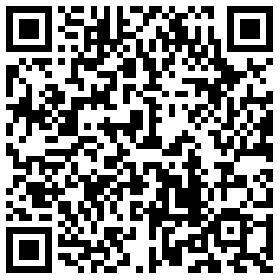# Double CT Solution

## 1. Introduction

The maximum rating of the our standard CT of the WiFi energy meters is 250A. If you want to measure the current larger than 250A, instead of using a large rating of CT with very large ratio, we recommend the more cost-effective solution with same measurement accuracy, double CT solution, which is also called Secondary Mutual Inductance.

## 2. Double CT Solution

As shown in below picture, taking 1000A as an example, if you want to measure the current up to 1000A, you can use two CTs, the primary CT and the secondary CT (the CT of our meter). The primary CT's rating is 1000A with the ratio 1000:5 (please select the ratio as xxx:5 because all our meters are calibrated with 5A current). The primary CT can be purchased by yourself. Clip the primary CT onto the live line to be measured. Then get a wire to link the primary CT's S1&S2 point together while clip the secondary CT onto the line between S1&S2 point.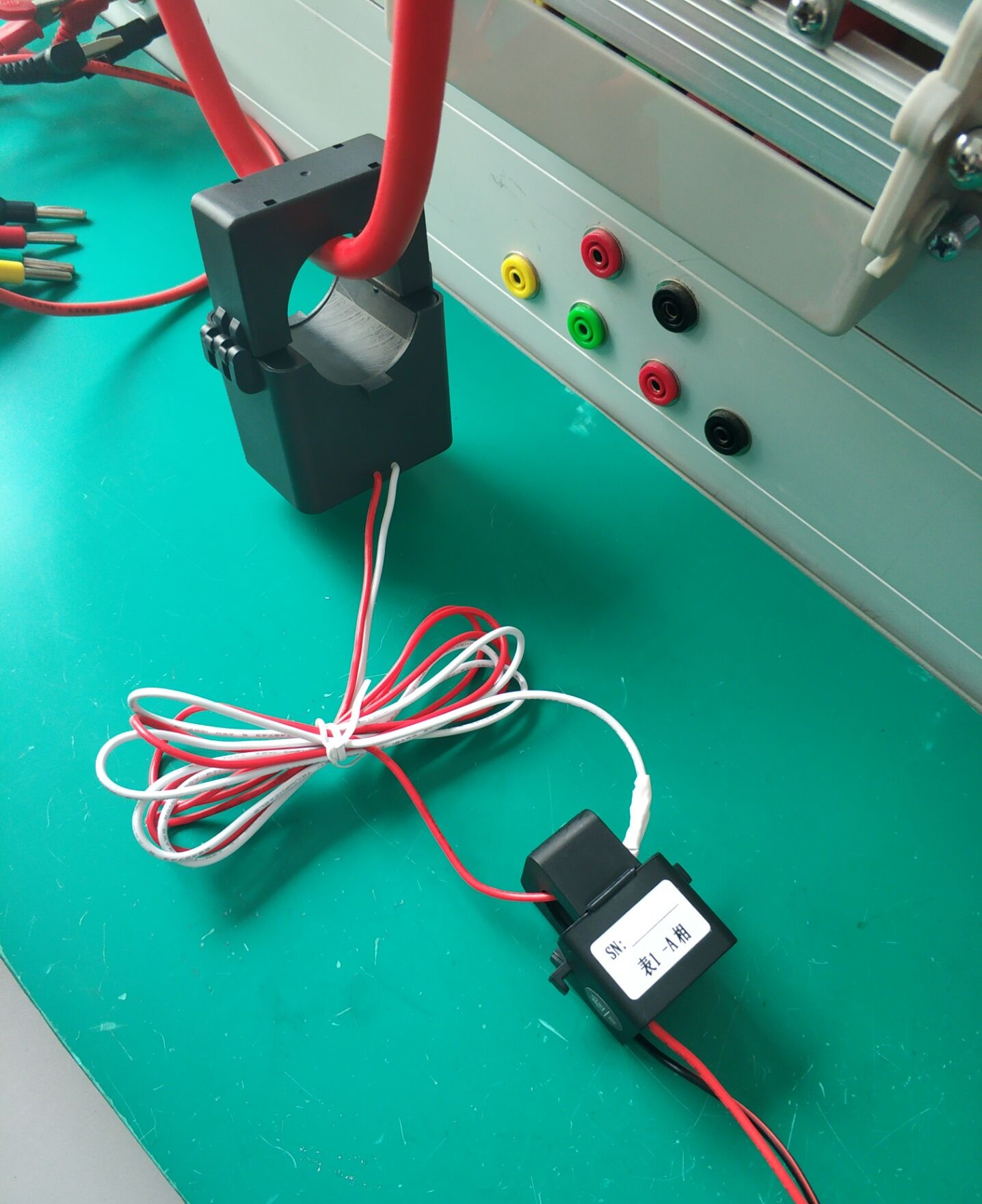The reading of the primary CT is the real value of the current, which is called Primary Mutual Inductance Current; The reading of the secondary CT is Secondary Mutual Inductance Current, the formula is:

Secondary Mutual Inductance Current = Real Current (Primary Mutual Inductance Current) / 200.

Tips: 200 = 1000:5, which is the ratio of primary CT

## 3. Set the CT ratio in Iammeter

As described above, still taking 1000A as an example, the reading reported to the cloud by our WiFi energy meter with secondary CT, is the Secondary Mutual Inductance Current. So you need to multiply it with 200 (1000:5) to get the real current value. So you need to configure this CT ratio (1000:5) in Iammeter and then the system will calculate the real value by multiplying the reported current readings with 200 (1000:5). And the system will also calculate the measurement which is related to current, such as active power, apparent power and energy by the same way.

Login to Iammeter and go to "Meters", then click "Edit" button on the meter. In the next "Edit Meter" webpage, you can select the "CT Ratio" according to the actual condition.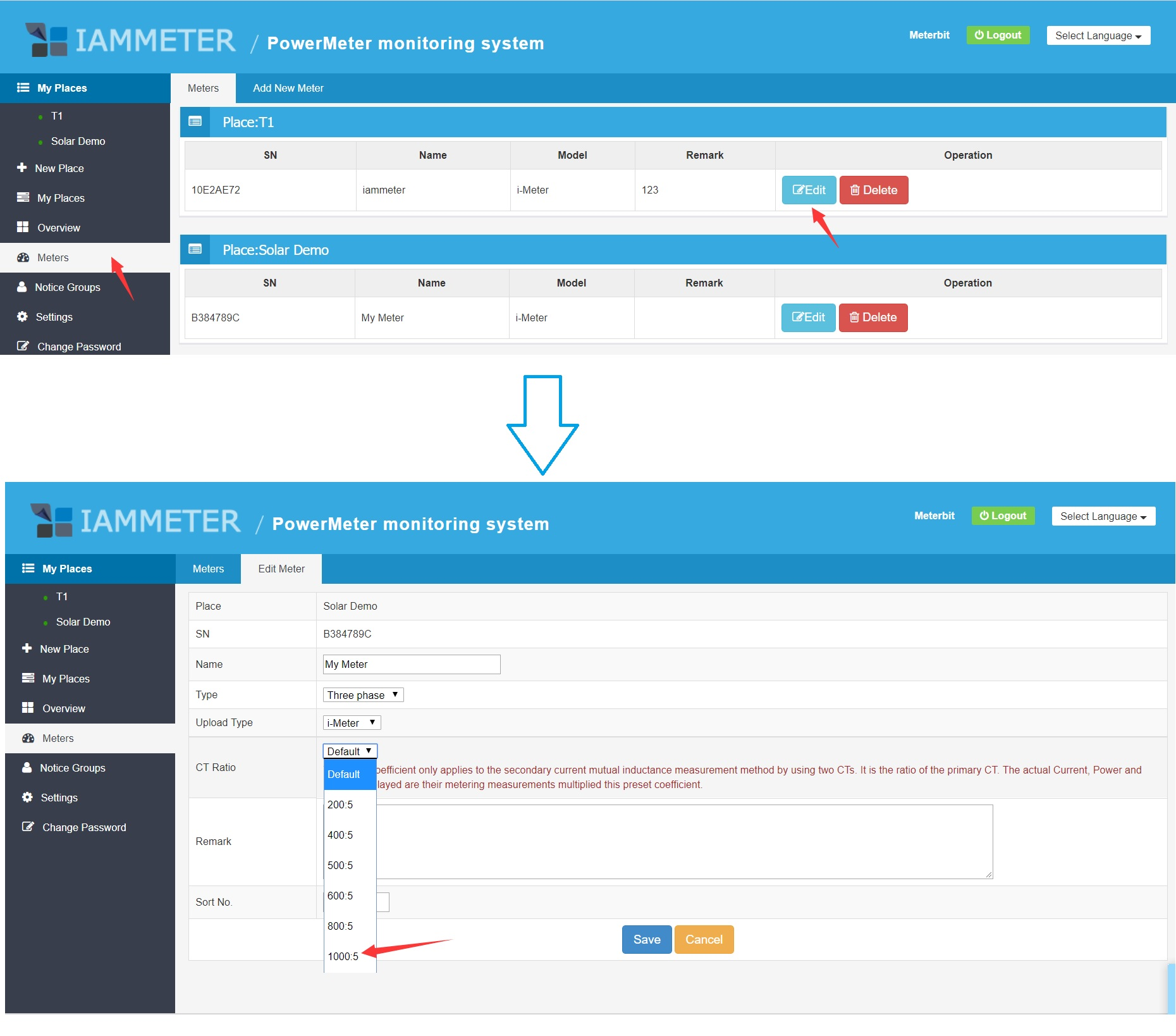### Note

If you have a WeChat account, please follow our IAMMETER WeChat public ID.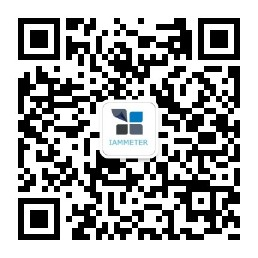## Contact

Contact me

•##### WhatsApp

+86 13911890238

•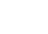##### Mail

support@devicebit.com

•##### WeChat Skype

+86 13911890238